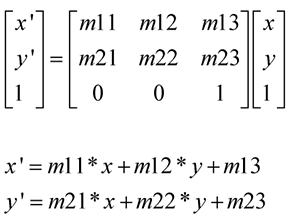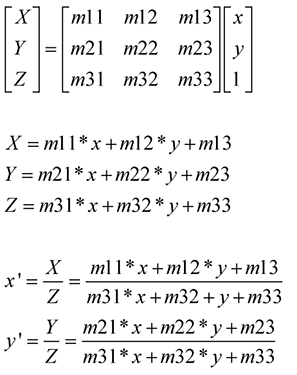图像处理之_仿射变换与透视变换

1.      仿射变换

2) 方法3) 举例

a) 以原点为中心旋转，2x3矩阵为：
[ cos(theta), -sin(theta), 0 ],
[ sin(theta), cos(theta), 0 ]

x’ = x * cos(theta) - sin(theta) * y
y’ = x * sin(theta) + cos(theta) * y

b) 平移，2x3矩阵为
[1,0,tx],
[0,1,ty]

x’ = x * 1 + y * 0 + tx = x + tx
y’ = x * 0 + y * 1 + ty = y + ty

4) 具体应用2.      透视变换（投影变换）

2) 方法3) 具体应用